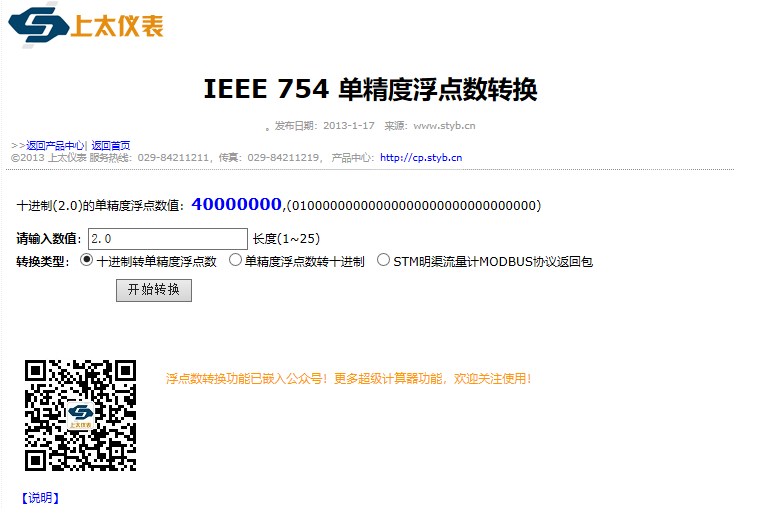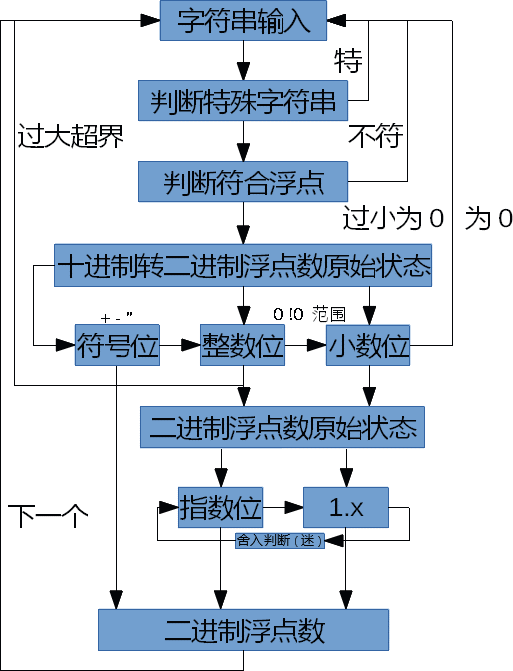• IEEE 754 单精度浮点数转换
万次阅读
2019-11-07 13:34:02

浮点型数据通讯的时候，需要将浮点数转成对应的四字节内存数据，可以通过该网址工具验证。

# IEEE 754 单精度浮点数转换

http://www.styb.cn/cms/ieee_754.php

1、IEEE754是由IEEE制定的有关浮点数的工业标准，其公式为：
X = (-1)S * 1.M * 2P-127
其中针对于单精度浮点数，S为符号位，只占1位，为0表示正数，为1表示负数。P为指数（阶码），用移码表示，占8位。M为尾数，用原码表示，占23位。

2、浮点数规格化表示：当尾数的值不为0时，规定尾数域的最高有效位为1，不符合规定的则将阶码左移或右移小数点位置。

原文链接：https://blog.csdn.net/qq_34801642/article/details/89183919

更多相关内容
• 绿色软件，免安装，解压即可使用。单精度浮点数转换工具
• 单精度浮点数转换程序vc6版本，程序很简单，就几个函数，很实用。
• 基于C++ Builder 6.0的IEEE 754中单精度浮点数转换实现研究论文
• 单精度浮点数转换器 实测功能OK 链接地址：http://www.styb.cn/cms/ieee_754.php

## 单精度浮点数转换器

第一种十进制数与浮点数转换器

功能1：十进制数转换为浮点数

功能2：单精度浮点数转换为十进制数

实测功能OK第一种十进制数与浮点数转换器

功能1：十进制数转换为浮点数

功能2：单精度浮点数转换为十进制数

实测功能OK上图：转换器小工具截图

展开全文• 浮点数和十六进制数的相互转换，包括：1.单精度浮点数（32位）和十六进制数的相互转换，2.双精度浮点数（64位）和十六进制数的相互转换
• num = str(input("输入一个数，显示其浮点数在二进制中的存储，输入exit退出：")) if num=="exit":#此处几个if是float定义的几个量 print("退出运算") break if num=="0":#此处几个if是float定义的几个量 ...

# 浮点数存储

十进制浮点数转换为二进制浮点数：整数部分重复相除，小数部分重复相乘
整数部分重复相除：除基取余，直到商为0，余数反转
小数部分重复相乘：乘基取整，直到小数为0或者达到指定精度位，整数顺序排列
匠心之作java基础强化之强转溢出&浮点数运算精讲

0.5
过程1：转换乘对应的二进制小数 0.1
过程2：转换成对应的指数形式 2**1 * 1.0

过程1分解，因为这里整数部分为0不用处理，相当于只需要了解怎么把十进制的.5变成二进制的.1，在人的思维中，这里的1就相当于2**-1，正好是0.5，但是这对计算机来说是没有意义的，计算机的运算器都是整数的运算，再观察，假设目标形态是x，0.5 * 2=1；x * 2=1，于是bin(0.1) is float(0.5)，那么如何用0.5推导出0.1，* 2对二进制意味着是比特左移，而小数点左边，对于计算机来说，是不认识小数的，于是只能当作整数处理，那么小数点左边这个整数就是5，5 * 2=10，即0.5 * 2=1，所以没假设的整数结果大于10后，就把这个十位拿掉存放起来，例如5 * 2一次就得到一个十位的1，拿掉1后就是0，获取小数结束：.1
那么为了知道规律，设定数值：bin(.11)即2**-1+2**-2即0.5+0.25即0.75

这里整数部分就是两位了，把这两个数当作整数就是75，那么要获取有效的1只能是百位：
75 * 2=150，拿掉百位的1，存储起来，待处理整数为50，50 * 2=100，拿掉百位的1，待处理数归零，取值结束，连续的获取了两个1，即bin(.11)
第一次拿掉的1，他表示的是0.5，第二次拿掉的1表示的是0.25，对应.11，第二次产生的1是放在第一次产生的1的后面。

假设我们不拿掉1，是否会有影响，150*2=300，个位、十位归零，运算结束，得到百位以上的数是3，3可以直接可以用二进制的数11表示，貌似也行。
方法1对应方法2的逻辑上的缺点：
1、貌似每次都要把超位的1都拿下来，这一步可以省去吗？
2、需要存储拿下来的1

优点：
1、超位后，拿掉一个位，之后就能少算一个位，于是每发生一次，之后运算压力都会减小，尤其是如果给一个小数点后很长数值的情况
2、接上，我们要每次都要推测超位的长度，因为我们规定了浮点数的数据存储长度就是那么多，存储长度盛满后，后面可能有的小数就要省略。而如果把位拿下来存在个东西里面，就只需要记录东西里面的长度。其实这里我很不确定，例如我用Python来表示，我会用到这个那个函数，但是我不知道机器中，是否有超位的部分的对应二进制的长度的运算方法。其实推测下来，可能的情况是待处理的数据其实是字符串，因为长度不定，计算机底层可能还无法存放那么长的数据，所以可能会需要用软件分割处理，所以不是单纯的数值，而数值只是计算的中间产物，而之后存放的二进制1，也是字符串，即：待处理字符串 == > 转换成运算器能处理的长度 == > 运算 == > 得到超位 == > 存放至表示二进制的字符串中。

上面提到的是小数部分如何转化成二进制小数部分，那么整数部分，其实就可以用十进制转二进制的方法直接转化成二进制整数部分。

Python 十进制转二进制

但是当作一个模拟硬件工作的流程，整数的0~9以及个十百千又如何变成二进制保存和运算的呢。保存其实就是一串二进制的01的串而已，没有类型规定的话，就是RAW。
又要开始思考了，假设我们拿到一个功能，即二进制1000就直接对应8，再底层的功能我们不考虑。
那么64位的cpu，他一次运算的长度可以是1111…挺长的，那就短说，假设我们用的处理器是4004，一次运算长度是4bit，即1111，从左往右，1分别对应8、4、2、1，最大表示无符号十进制15，假设给出整数0~9的任意一个，例如8，他记住了bin(1000)是8，结果就直接出来了。例如7，他至少知道7比8小，比4大，所以7-4=3，同理3-2=1，于是0111，当然他也可能直接记住7是0111。那么给出16呢，超出15的界限，这才开始真正的思考而不是推测+思考。16是一个字符串，他符合整数的书写规则，而且我们也是要把他当作整数处理。底层库中并没有他的数据，但是运算器中有3/2=1…1；5/2=2…1；6/2=3；7/2=3…1；9/2=4…1的数据，16就是一个十位的1和一个个位的6，16/2=（10+6）/2=5+3=8，而8正好是1000，而十进制的/2运算就是二进制的位移运算，所以16的二进制形态就是10000，那么17呢，17/2=（10+7）/2=8…1，余号前面意味着仍有10000，但余数意味着之前有个1，那么就是10000+1=10001。貌似有了苗头，只需要定义一个10/2=5。
那么一个更大点更复杂的数，例如bin(111111)，int(0b111111): 63。求63在4004下转成二进制形态的过程。
5/2=2…1 : 1 2/2=1…0 : 0 1/2=0…1 : 1 5:bin(101)

bin(1010):10 10/2=5…0 : 0 5 : 101 越开始的位，越是二进制的低位（右侧）

    s=str(i%2)+s
i=i//2

>>>s '1010011000' 缕一缕思路，只要整数/2，无论整除还是有余，得到的结果相对于二进制来说，都是上了一位。而余数就是当前位置二进制的单位。无注释版：

import re

while 1:
num = str(input("输入一个数，显示其浮点数在二进制中的存储，输入exit退出："))
if num=="exit":
print("退出运算")
break
if num=="0":
print("00000000000000000000000000000000")
continue
if num=="nan":
print("01111111110000000000000000000000")
continue
if num=="inf":
print("01111111100000000000000000000000")
continue
if num=="-inf":
print("11111111100000000000000000000000")
continue

if ((not re.compile("[^0-9.\-+]+").findall(num)) \
and re.compile("[0-9]+").findall(num) \
and ((num.count("-") == 0 and num.count("+") == 0) \
or (num.count("+") == 0 and num.count("-") == 1 and num == "-") \
or (num.count("-") == 0 and num.count("+") == 1 and num == "+")) \
and (num.count(".") == 0 or num.count(".") == 1)):

if num=="-":
sign="1"
num=num[1:]
elif num=="+":
sign="0"
num = num[1:]
else:sign="0"

if num.count("."):
numi=num[0:num.find(".")]
numm=num[num.find(".")+1:]
else:
numi = num
numm = "0"

if numi=="0" and numm=="0":
print("00000000000000000000000000000000")
continue

numib=bin(int(numi if numi else "0"))[2:]

if numm!="0":
nummb=""
top=10**len(numm)
numm=int(numm)
if numib != "0":
lmb=(25-len(numib))
while len(nummb)<lmb:
numm=numm*2
if numm>top:
nummb+="1"
numm-=top
elif numm==top:
nummb += "1"
break
else:nummb += "0"
else:
for i in range(126):
numm = numm * 2
if numm<top:nummb += "0"
elif numm>top:
nummb += "1"
numm -= top
for i in range(24):
numm = numm * 2
if numm > top:
nummb += "1"
numm -= top
elif numm == top:
nummb += "1"
break
else:
nummb += "0"
break
elif numm==top:
nummb += "1"
break
if "1" not in nummb:
print("数值过小，赋值为0\n00000000000000000000000000000000")
continue
else:nummb="0"

numb=numib+"."+nummb

nume=numb.find(".")-1 if numb.find("1")<numb.find(".") else -(numb.find("1")-1)
print(numb)
if nume>127:
print("数值过大，超出范围")
continue
exponent=bin(nume+127)[2:].rjust(8,"0")

mantissa=numb[:numb.find(".")]+numb[numb.find(".")+1:]
mantissa=mantissa.lstrip("0")
print(bin(nume+127))

if sign=="0" and len(mantissa)==25 and mantissa=="1":
mantissa=bin(int(mantissa[:25],2)+1)[2:]
if len(mantissa)==26:
exponent=bin(nume+127+1)[2:].rjust(8,"0")

if len(mantissa)<25:print("该浮点数精确无舍入")
else:print("该浮点数不精确有舍入")

mantissa=mantissa[1:24].ljust(23,"0")
numf=sign+exponent+mantissa
print(numf)

else:
print("格式错误，无法转换为浮点数")


注释版：

import re

# 首先我们要知道在没有软硬件支持浮点数的那个时代，先贤们是怎么定义了单精度浮点数
# 并利用那时的条件运算出来。经过多次改良优化后，我攒出了以下代码
# 首先要了解单精度浮点数：32位的存储长度，其中第一位存储正负号：sign bit
# 接下来的八位存储指数：2**E，exponent，8位二进制范围是0~256，但因为之后
# 定义基数为1.x的原因，所以E要适应有效数字在小数点前咋滴咋滴，小数点后咋滴咋滴
# 所以将01111111，即127作为基准位0。所以E的有效范围是-127~128，但又因为在浮点系统中
# 定义了几个特殊的项，所以还是占用了几个E，大家可以用struct玩玩看
# 基数，1.x，在浮点数的二进制中，1会被隐去。.x的二进制占23位。python与IEEE754
# 在最后位除非被其舍去的下一位有分歧，python是0舍1入。IEEE754是直接截断。（可能）
# 所以我在代码中做了第25位的判断 /嘿嘿嘿 又优化了运算过程，并给出数值是否被舍入
while 1:
num = str(input("输入一个数，显示其浮点数在二进制中的存储，输入exit退出："))
if num=="exit":#此处几个if是float定义的几个量
print("退出运算")
break
if num=="0":#此处几个if是float定义的几个量
print("00000000000000000000000000000000")
continue
if num=="nan":#没有数
print("01111111110000000000000000000000")
continue
if num=="inf":#无穷大
print("01111111100000000000000000000000")
continue
if num=="-inf":#无穷小
print("11111111100000000000000000000000")
continue

#检测字符串是否符合浮点数规格
if ((not re.compile("[^0-9.\-+]+").findall(num)) \
and re.compile("[0-9]+").findall(num) \
and ((num.count("-") == 0 and num.count("+") == 0) \
or (num.count("+") == 0 and num.count("-") == 1 and num == "-") \
or (num.count("-") == 0 and num.count("+") == 1 and num == "+")) \
and (num.count(".") == 0 or num.count(".") == 1)):

#判断正负
if num=="-":
sign="1"
num=num[1:]
elif num=="+":
sign="0"
num = num[1:]
else:sign="0"

#分离整数部分和小数部分
if num.count("."):
numi=num[0:num.find(".")]
numm=num[num.find(".")+1:]
else:
numi = num
numm = "0"

if numi=="0" and numm=="0":
print("00000000000000000000000000000000")
continue

#整数部分的二进制
# 虽然这里我用了bin直接转换整数为二进制，但是实际上
# 那个年代使用的整数是多少位的，因为浮点数最大支持3.x * 10**38
# 要远比int大的多
numib=bin(int(numi if numi else "0"))[2:]

#小数部分的二进制
#如果numm不为0，进行运算，else，直接赋值nummb="0"
if numm!="0":
#存储二进制的字符串
nummb=""
#作为比较的基数，之后底数*2，二进制的意思就是向左位移1
# 等一等，那我岂不是，冷静下，不是这样的，因为这里要算出
# 对应的浮点数的小数点二进制部分，而此时根本不知道小数点
# 二进制部分如何表示，这里就是要运算出来，而不是单纯的
# 向左位移，而top这个就想到与二进制中的1的位置，而小数点
# 后的二进制则是黑盒，现在就是要用*2，即黑盒中的现在不知
# 但以后确实能存在的二进制浮点数给推出来
top=10**len(numm)
numm=int(numm)
#如果整数部分存在数值，先贤们用32位定义为单精度浮点数
# 经过指数运算后最终留给1.x的.x表示长度是23个二进制位
#为了节省运算效率，不做多余的一丁点的运算，所以这里分叉了
# 未优化前可是算152次的 127+25
if numib != "0":
lmb=(25-len(numib))
while len(nummb)<lmb:
# *2相当于<<1
# 这里就是要把小数点后的二进制排列给挤出来
numm=numm*2
if numm>top:
nummb+="1"
numm-=top

#之前写过一个代码，有类似的过程，因为预先知道
# 之后不会有东西了，锁了舍去了num-的过程
# 直接跳出，但之后有用到num==0的判断，所以
# 这里激灵了一下，大概之后用不到numm了吧！？
elif numm==top:
nummb += "1"
break
else:nummb += "0"
else:
# 这个岔路，是整数部分为0的情况，所以1.x的位置很不确定
# 所以就开始算，直到第一个有效数字出现，然后再算24个数字
# 之前说过无脑152，但是这里简化以下，如果在e的最大值126下
# 仍然无法得到有效数值，就直接赋值为0
for i in range(126):
numm = numm * 2
if numm<top:nummb += "0"
elif numm>top:
nummb += "1"
numm -= top
for i in range(24):
numm = numm * 2
if numm > top:
nummb += "1"
numm -= top
elif numm == top:
nummb += "1"
break
else:
nummb += "0"
break
elif numm==top:
nummb += "1"
break
# if nummb.find("1") == -1:
# 文档说了，判断有没有用in，不用find
if "1" not in nummb:
print("数值过小，赋值为0\n00000000000000000000000000000000")
continue
else:nummb="0"

#原始过程中的浮点数二进制不带正负号
numb=numib+"."+nummb

#原始二进制浮点转换成 1.x*(2**E) 计算2**E，指数
nume=numb.find(".")-1 if numb.find("1")<numb.find(".") else -(numb.find("1")-1)
print(numb)
if nume>127:
print("数值过大，超出范围")
continue
exponent=bin(nume+127)[2:].rjust(8,"0")

#规整1.x
mantissa=numb[:numb.find(".")]+numb[numb.find(".")+1:]
mantissa=mantissa.lstrip("0")
print(bin(nume+127))
#判断是否需要入尾位，这个是python的特殊定义，IEEE 754 单精度浮点数转换并无舍入

if sign=="0" and len(mantissa)==25 and mantissa=="1":
mantissa=bin(int(mantissa[:25],2)+1)[2:]
if len(mantissa)==26:
exponent=bin(nume+127+1)[2:].rjust(8,"0")

if len(mantissa)<25:print("该浮点数精确无舍入")
else:print("该浮点数不精确有舍入")
#拼接浮点二进制
mantissa=mantissa[1:24].ljust(23,"0")
numf=sign+exponent+mantissa
print(numf)

else:
print("格式错误，无法转换为浮点数")


以上是第三，不，第四天的码了，准确的说是从想写一个码开始，第五天了。这几天没什么学习进度，心里挺焦急的！

而实际上python默认并不会用到单精度浮点数，所以struct中的末位0舍1入到底是怎样，不的而知。
看看python实际上用的双精度浮点数吧！

In : struct.unpack("!d",b'\x3f\xff\xff\xff\xff\xff\xff\xff')
Out: (1.9999999999999998,)

In : struct.pack("!d",1.9999999999999998)
Out: b'?\xff\xff\xff\xff\xff\xff\xff'

In : 2**-53
Out: 1.1102230246251565e-16

In : struct.pack("!d",1.99999999999999986)
Out: b'?\xff\xff\xff\xff\xff\xff\xff'

In : struct.pack("!d",1.99999999999999987)
Out: b'?\xff\xff\xff\xff\xff\xff\xff'

In : struct.pack("!d",1.99999999999999988)
Out: b'?\xff\xff\xff\xff\xff\xff\xff'

In : struct.pack("!d",1.99999999999999989)
Out: b'@\x00\x00\x00\x00\x00\x00\x00'

In : struct.unpack("!d",b'@\x00\x00\x00\x00\x00\x00\x00')
Out: (2.0,)

In : a=1.99999999999999989

In : a
Out: 2.0

In : a=1.99999999999999988

In : a
Out: 1.9999999999999998


我要放弃思考

=============================================================================

两天，没白费，期间搞出好多问题，现在接近于当初还没有浮点软硬件支持时，前辈大佬们的想法了吧！

第二版，参照了前辈的代码思路 \心痛.png

import re

while 1:
num = str(input("输入一个数，显示其浮点数在二进制中的存储，输入exit退出："))
if num=="exit":#此处几个if是float定义的几个量
print("退出运算")
break
if num=="0":#此处几个if是float定义的几个量
print("00000000000000000000000000000000")
continue
if num=="nan":#没有数
print("01111111110000000000000000000000")
continue
if num=="inf":#无穷大
print("01111111100000000000000000000000")
continue
if num=="-inf":#无穷小
print("11111111100000000000000000000000")
continue

#检测字符串是否符合浮点数规格
if ((not re.compile("[^0-9.\-+]+").findall(num)) \
and re.compile("[0-9]+").findall(num) \
and ((num.count("-") == 0 and num.count("+") == 0) \
or (num.count("+") == 0 and num.count("-") == 1 and num == "-") \
or (num.count("-") == 0 and num.count("+") == 1 and num == "+")) \
and (num.count(".") == 0 or num.count(".") == 1)):

#判断正负
if num=="-":
sign="1"
num=num[1:]
else:sign="0"

#分离整数部分和小数部分
if num.count("."):
numi=num[0:num.find(".")]
numm=num[num.find(".")+1:]
else:
numi = num
numm = "0"

#整数部分的二进制
numib=bin(int(numi if numi else "0"))[2:]

#小数部分的二进制
if numm:
nummb=""
top=10**len(numm)
numm=int(numm)
print(numm)
while len(nummb)<152:
numm=numm*2
if numm>=top:
nummb+="1"
numm-=top
else:nummb+="0"
else:nummb="0"

#原始过程中的浮点数二进制不带正负号
numb=numib+"."+nummb

#原始二进制浮点转换成 1.x*(2**E) 计算2**E，指数
nume=numb.find(".")-1 if numb.find("1")<numb.find(".") else -(numb.find("1")-1)
exponent=bin(nume+127)[2:].rjust(8,"0")

#规整1.x
mantissa=numb[:numb.find(".")]+numb[numb.find(".")+1:]
mantissa=mantissa.lstrip("0")

#判断是否需要入尾位，这个是python的特殊定义，IEEE 754 单精度浮点数转换并无舍入
if sign=="0" and mantissa=="1":
mantissa=bin(int(mantissa[:25],2)+1)[2:]
if len(mantissa)==26:
exponent=bin(nume+127+1)[2:].rjust(8,"0")

#拼接浮点二进制
mantissa=mantissa[1:24].ljust(23,"0")
numf=sign+exponent+mantissa
print(numf)

else:
print("格式错误，无法转换为浮点数")


参考：Python实现十进制小数转IEEE754单精度浮点数转换
参考中的代码有一次bug，是因为引入了浮点运算，浮点运算的舍入和内部显示导致结果会出现偏差甚至错误。
我改进了下，以至于以上代码是纯整数运算，并添加了python的舍入机制。

之前搞的错误思路的代码，贴在下边，删除以前的错误文章！

python - 浮点数的存储结构 一笔笔教你怎么算

我犯了个错误，本想用一段代码表示浮点的转换存储，但是里面却不断的使用着与浮点相关的运算，如果这几一个实用的东西的话，她就是个死循环。
1、一开始赋值就转换成float，这导致之后接受的都是转换后的数据，大几率的错误性
例如，了解浮点存储的，再去想最大浮点值
0 11111110 11111111111111111111111
+ 2**127 *1.99999999…=3.402823669209385e+38
我就想到0.9999999999999…这个很边缘的值，他在浮点M值以外的位舍入后，就是1.0了，而float就是这样干的
在此之前我就针对进位做了个运算了，但用0.9999999999999…验证时，才注意到了最初我就犯了错误。
本想着再模拟486以前的没有浮点机制的时候去写个。思路很难，即便完成了指数的取值，小数部分的取值又要涉及到浮点运算。
而且浮点之所以这么存储，大概也是为了做浮点间的运算的方便，之后就是一个个很深的水了，于是虽然不想放弃的我，放弃这个问题，赶紧学下去才是关键吧！至于那个往上的在线运算器，会不会就是利用struct写的，毕竟就是一句话的事！即便也是用输入是字符串，解析成浮点存储结构，也不难，但如果真的没有利用一点浮点运算，大家能写出来吗？

IEEE 754 单精度浮点数转换
我嗅探了下网络，这个运算是联网的，网址所在地：美国俄勒冈波特兰 亚马逊云

不过我还是想尝试下至少一开始是字符输入接收为字符串的方法

=======================================================
这段代码并不完善，但大家可以先看看，完善的代码在后面。

第一版：用的是算数的思路，自己啃书，磕出来的代码，在纸上算的话，可以说是完美，但是在电脑上算，涉及到了好多那个时代不该出现的浮点运算，甚至最开始输入的字符串转化时，就是转化成了浮点数，最关键的是他可能会发生舍入导致结果不是我们输入的那个结果，所以虽然是一个纸上的完美思路。但是实际仅有教学价值了吧！

while 1:
try:#如果输入转化失败，激发之后的except:break，结束程序
num=float(input("请输入一个浮点数，非数结束："))
except:break
if num==0:#此处几个if是float定义的几个量
print("00000000000000000000000000000000")
continue
if num=="nan":#没有数
print("01111111110000000000000000000000")
continue
if num=="inf":#无穷大
print("01111111100000000000000000000000")
continue
if num=="-inf":#无穷小
print("11111111100000000000000000000000")
continue

# 判断符号位 S：sign bit
sign= "0" if num>0 else "1" #简化后的代码
# if num>0:sign="0"
# else:sign="1"

# 推算指数 E:exponent 指数
# 将数累计计算直到1.x的格式，一切都是二进制运算
e=0
if num>=2:
while num>=2:
num/=2
e+=1
elif num<1:
while num<1:
num*=2
e-=1
#以上累计的e
exponent=bin(e+127).replace("0b","").rjust(8,"0")

# 推算尾数 M:mantissa 尾数、小数部分
num-=1
if num==0:
print(sign+exponent+"00000000000000000000000")
continue
mantissalist=[]
#这里我一开始是使用字符串的，但是最后一位判断的原因，
# 字符串无法修改，所以做成了列表
for i in range(1,24):
b=2**-i
if num>b:
num-=b
mantissalist.append("1")
elif num==b:
num -= b
mantissalist.append("1")
break
elif num<b:
mantissalist.append("0")
# print(i,num==0) #debug时增加的输出

# 判断是否计算到最后位还有余数，余数是否大于等于无法显示的那位
# 如果是，舍入结果是可显示的最后一位是1，这里我无法断定如果等于
# 的话会怎样因为根本无法把正好等于的那个数打进去，但八成就是这样
if i==23 and mantissalist=="0" and num>=2**-24:
mantissalist="1"
mantissa=''.join(mantissalist).ljust(23,"0")
print(sign+exponent+mantissa)


以上是用python模拟的float存储方式，大家可以对应系统自带的一个库算出的结果对照，但是那个库貌似也不是真实存储状态，反正差不多！
这个是调用struct模块，返回各种效果，数据准确，大概。就是这里，给出的结果是0舍1入，至于python实际，要不咱算算？

import struct
# def binary(num):
#     return ''.join(bin(ord(c)).replace('0b', '').rjust(8, '0') for c in struct.pack('!f', num))
# 上面注释掉的是源代码，总是报错
while 1:
try:a=float(input("输入一个数，他会输出对应的浮点数结构，输入非数类退出："))
except:break
else:
print(''.join(bin(h).replace('0b','').rjust(8,'0') for h in struct.pack('!f',a)))

# 这里是我在写模拟过程时，用的0.0456与最后一位何时0何时1调用的几个参数
# print(struct.pack('!f',0.04559999704360962))
# print(struct.unpack('!f',b'=\xcc\xcc\xcd'))
# print(struct.unpack('!f',b'=:\xc7\x11'))
# print(struct.unpack('!f',b'=:\xc7\x10'))


使用unpack得到：
01110101100011100010001 0.04560000076889992
01110101100011100010000 0.04559999704360962
最后一位1，0.00000000372529030
0.04560000263154507

In : struct.pack("!f",0.0455999979749322)
Out: b'=:\xc7\x10'

In : struct.pack("!f",0.04560000263154507)
Out: b'=:\xc7\x12'

In : struct.pack("!f",0.04560000263154506)
Out: b'=:\xc7\x11'


额，不行，这个舍入无视末位0还是1，只要进位，可能会一串上去
还需要改

==========================================
额，暂时算法上搞定了，但是突然觉得这里从字符串该过来的列表又没必要了，所以再简化成字符串吧！

while 1:
try:#如果输入转化失败，激发之后的except:break，结束程序
num=float(input("请输入一个浮点数，非数结束："))
except:break
if num==0:#此处几个if是float定义的几个量
print("00000000000000000000000000000000")
continue
if num=="nan":#没有数
print("01111111110000000000000000000000")
continue
if num=="inf":#无穷大
print("01111111100000000000000000000000")
continue
if num=="-inf":#无穷小
print("11111111100000000000000000000000")
continue

# 判断符号位 S：sign bit
sign= "0" if num>0 else "1" #简化后的代码
# if num>0:sign="0"
# else:sign="1"

# 推算指数 E:exponent 指数
# 将数累计计算直到1.x的格式，一切都是二进制运算
e=0
if num>=2:
while num>=2:
num/=2
e+=1
elif num<1:
while num<1:
num*=2
e-=1
#以上累计的e
exponent=bin(e+127).replace("0b","").rjust(8,"0")

# 推算尾数 M:mantissa 尾数、小数部分
num-=1
if num==0:
print(sign+exponent+"00000000000000000000000")
continue
mantissalist=[]
#这里我一开始是使用字符串的，但是最后一位判断的原因，
# 字符串无法修改，所以做成了列表
for i in range(1,24):
b=2**-i
if num>b:
num-=b
mantissalist.append("1")
elif num==b:
num -= b
mantissalist.append("1")
break
elif num<b:
mantissalist.append("0")
# print(i,num==0) #debug时增加的输出

# 判断是否计算到最后位还有余数，余数是否大于等于无法显示的那位
# 如果是，舍入结果是可显示的最后一位是1，这里我无法断定如果等于
# 的话会怎样因为根本无法把正好等于的那个数打进去，但八成就是这样
if i==23 and num>=2**-24:
# exec("mantissa=(bin(int(%s)+1).replace('0b','').rjust(23,'0')[-1:-24:-1][::-1])"%('0b'+''.join(mantissalist)))
mantissa=(bin(int('0b'+''.join(mantissalist),2)+1).replace('0b','').rjust(23,'0')[-1:-24:-1][::-1])
else:mantissa=''.join(mantissalist).ljust(23,"0")
print(sign+exponent+mantissa)


还是有毛病，致命的大毛病，我获取的num本身其实就是个float，所以我不能使用a=float(input())。
而是要用a=str()，然后判断他是否符合录入对像的规则。

因为我录入0.9999999999999999999999，模拟她肯定接近于1，所以她的存储和1是一样的，于是struct是的，但是我的也是的，但我的不该是呀！所以错了！

请输入一个浮点数，非数结束：0.0456
0.0456
01111010
00111101001110101100011100010001
请输入一个浮点数，非数结束：0.99999999999999999999999999999999
1.0
01111111
00111111100000000000000000000000
`

于是我又加了个print，果然如此，所以继续改！亏的看了，不然真就错了！看来我注定不是一个码农，而是一个自由者！

展开全文• 单精度精度浮点数转换，浮点数与16进制转换工具
• 纯汇编编写的浮点数转换工具，是汇编编程的必备工具
• 该工具为单精度与双精度的浮点转换成十进制，或者十进制转换单精度或双精度的工具，可以验证你的计算方法是否错误。
• 单精度浮点数与十六进制转换_C语言程序__单片机也可用
• 单精度浮点数转十进制方法一、单精度浮点数符合IEEE754标准，32位，前面第一位是符号位，接下来的8位是指数，最后23位是尾数。编程中了解这些就够了，转换方法如下：二、VB中转换示例：'VB浮点数转换程序Option ...

单精度浮点数转十进制方法

一、单精度浮点数符合IEEE754标准，32位，前面第一位是符号位，接下来的8位是指数，最后23位是尾数。编程中了解这些就够了，转换方法如下：

二、VB中转换示例：

'VB浮点数转换程序

Option Explicit       '利用函数CopyMemory转换

Private Declare Sub CopyMemory Lib "kernel32" Alias "RtlMoveMemory" (Destination As Any, Source As Any, ByVal Length As Long)

Dim F As Single       '十进制数

Dim A(3) As Byte      '16进制浮点数

Private Sub Command1_Click()          '转换为十进制数

A(0) = CLng("&H" & Text1(3).Text)   '16进制字符转数字

A(1) = CLng("&H" & Text1(2).Text)

A(2) = CLng("&H" & Text1(1).Text)

A(3) = CLng("&H" & Text1(0).Text)

CopyMemory F, A(0), 4               '转换

Text2.Text = F                      '显示结果

End Sub

Private Sub Command2_Click()          '转换为浮点数格式

F = Val(Text2.Text)                 '十进制字符转数字

CopyMemory A(0), F, 4              '转换

Text1(0).Text = IIf(A(3) > 15, Hex(A(3)), "0" & Hex(A(3)))  '显示结果

Text1(1).Text = IIf(A(2) > 15, Hex(A(2)), "0" & Hex(A(2)))

Text1(2).Text = IIf(A(1) > 15, Hex(A(1)), "0" & Hex(A(1)))

Text1(3).Text = IIf(A(0) > 15, Hex(A(0)), "0" & Hex(A(0)))

End Sub

三、C中转换示例：

union sf

{

float f;

unsigned char s;

}a;

float m;

unsigned char t

//转换为十进制数

a.s=0x51;  //低位在前

a.s=0x06;

a.s=0x9E;

a.s=0x3F;

m=a.f;

//转换为浮点数格式

a.f=m;

t=a.s;

t=a.s;

t=a.s;

t=a.s;

展开全文• 本代码将双精度浮点数转换单精度浮点数，适合浮点数为正值的转换。 使用后将占用VD2810~VD2970字节，欢迎交流。 本代码的完成经历了一段时间的刻苦研究，无偿提供给真正需要的人，希望同行少走弯路。 代码允许复制...
•c++ float
• 此模型说明了将单精度浮点数转换为等效的无符号 32 位字和 8 位（四字节）数据结构并返回的两种方法。 主要目的是提供几种不同的方法来处理 Simulink 中这种类型的转换和位级操作（而不是作为特定问题的综合指南或...matlab
• 工作中碰到的一个小问题，经过了一番研究，终于搞明白了，为了以后大家不再挠头，写了这个供大家参考。其中涉及到MODTEST 软件 MODBUS协议 IEEE32位二进制浮点数与十进制小数转换的方法等内容。
• 此文章描述了如何将十进制小数转换为二进制小数以及浮点数再内存中时如何进行存储。 二进制小数 在计算机中，无论什么数据，最终存储都为二进制，对于整数部分很容易表示，但是对于非整数则比较困难。例如，十进制数...数据结构
• 在十六进制数和单精度浮点数之间转换转换非常简单，容易上手，一般人想不到的方法，符合IEEE754协议。
• IEEE二进制浮点数算术标准（IEEE 754）是1980年代以来最广泛使用的浮点数运算标准，为许多CPU与浮点运算器所采用。这个标准定义了表示浮点数的格式（包括负零-0）与反常值（denormal... 本资源为IEEE-754单精度转换程序
• 如下为十进制小数转IEEE754标准的单精度浮点数： def ConvertFixedIntegerToComplement(fixedInterger) :#浮点数整数部分转换成补码(整数全部为正) return bin(fixedInterger)[2:] def ConvertFixedDecima.....计算机系统基础 Python
• 二进制 IEEE单精度浮点数转化为十进制浮点数 公式: （-1）s ×1. M ×2（E-127） 二进制IEEE单精度浮点数为32位， 第1位为阶符（sign）， 第2位至第9位为阶码（exponent）， 第10位至第32位为尾数（mantissa）。 S为...经验分享
• ## 双精度浮点数转换

热门讨论 2013-08-19 17:24:54
双精度浮点数、单精度浮点数与十六进制，二进制之间可以进行任意转换
• VB小工具，免费使用，实现float数据与四字节数据相互转化以及Double数据与八字节数据相互转化。
• 针对于单精度浮点数，其公式如下，S为符号位，只占1位，为0表示正数，为1表示负数。P为指数(阶码)，用移码表示，占8位。M为尾数，用原码表示，占23位。X = (-1)S * 1.M * 2P-127浮点数规格化表示：当尾数的值不为0时...
• #include<stdio.h> /*--------------------------- 十六进制到浮点数 ---------------------------*...unicode qt c语言
• 在做MODBUS通讯时经常会用到单精度浮点数来表示测量值，比如41 20 00 00代表十进制的10，用代码可以轻松实现转换，但是他是怎么计算出来的呢？查了一些资料后我知道了计算的方法，在此记录一下。 举个栗子： 比如10...modbus
• 单精度浮点数与二进制数之间转换IEEE754标准浮点数数与二进制数转换 IEEE754标准 IEEE二进制浮点数算术标准（IEEE 754） 规定了四种表示浮点数值的方式：单精确度（32位）、双精确度（64位）、延伸单精确度（43比特...
• 单精度浮点数的表示方法 三个主要成分是： Sign（1bit）：表示浮点数是正数还是负数。0表示正数，1表示负数 Exponent（8bits）：指数部分。类似于科学技术法中的M*10^N中的N，只不过这里是以2为底数而不是10。......